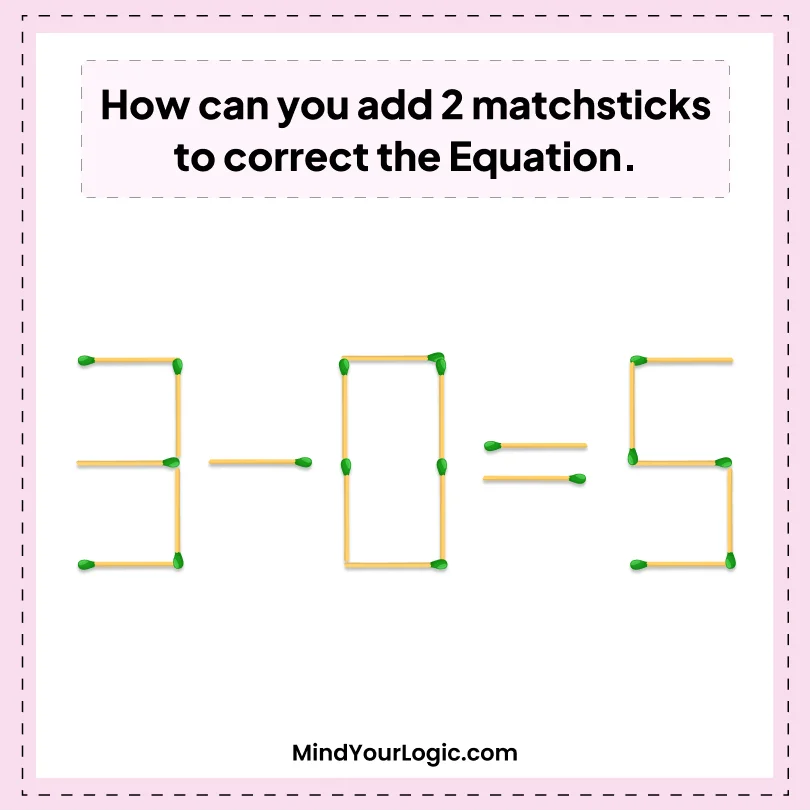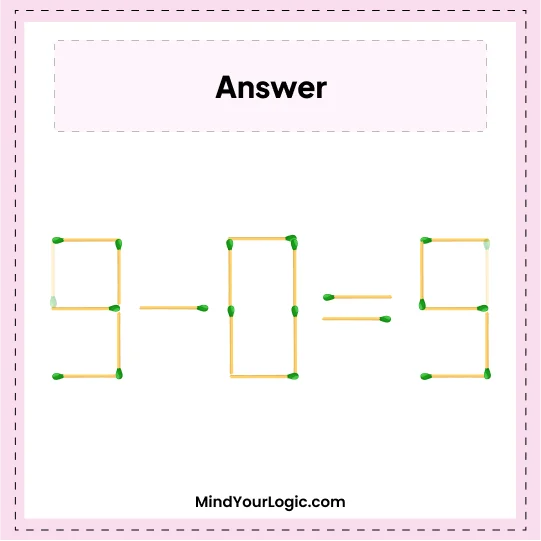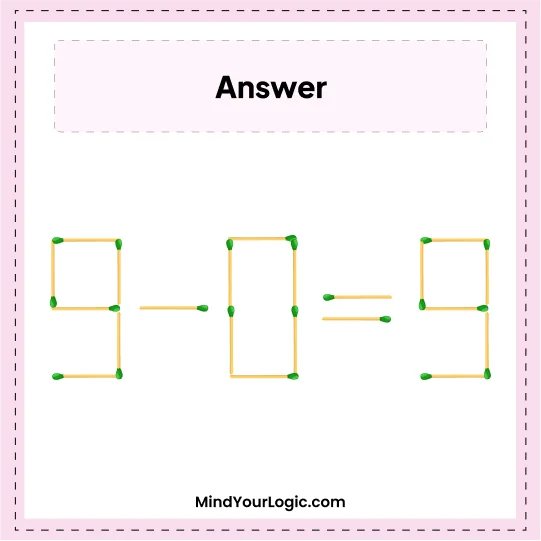# 3-5=0 - Equation Matchsticks Puzzle

###### 27.Matchstick Puzzles
`How Can You Add Two Matchsticks to Correct the Equation.`•

Explanation :

``` Let's add the sticks,
Add 1st matchstick to the 1st number 3 and make it 9.
Add 2nd matchstick to the last number 5 and make it into 9.
So, we get the correct equation as below : .
9-0=9
```